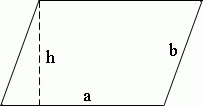Remove from favorites

# Area of a parallelogram, parallelogram area formula calculator

Area of a parallelogram, parallelogram area formula calculator allows you to find an area of a parallelogram, by the formulas, using length of parallelogram sides, height, diagonals and angle between them.

## Method of calculating the area of a parallelogram:

Side:    Height:Parallelogram is a quadrilateral with two pairs of parallel sides.
Formula for area of a parallelogram:,
where a - side of a parallelogram, h - height